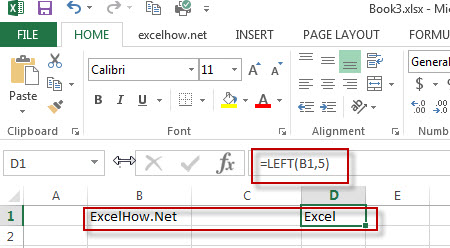# Excel Left Function

This post will guide you how to use Excel LEFT function with syntax and examples in Microsoft excel.

### Description

The Excel LEFT function returns a substring (a specified number of the characters) from a text string, starting from the leftmost character.

The LEFT function is a build-in function in Microsoft Excel and it is categorized as a Text Function.

The LEFT function is available in Excel 2016, Excel 2013, Excel 2010, Excel 2007, Excel 2003, Excel XP, Excel 2000, Excel 2011 for Mac.

### Syntax

The syntax of the LEFT function is as below:

= LEFT(text,[num_chars])

Where the LEFT function arguments are:
Text -This is a required argument. The text string that you want to extract from.
num_chars-This is an optional argument. It will specify the number of characters that you want to extract from Text string.

Note: The Num_chars value must be greater than or equal to zero. If Num_chars is greather that the length of text string, it will return all of text string. If Num_chars value is omitted, it is set to be 1 by default.

### Example

The below examples will show you how to use Excel LEFT Text function to extract the leftmost substring from a text string.

#1 To extract the leftmost 4 characters in the B1 cell, just using formula: =LEFT(B1,4).### More LEFT Function Examples

• Split Text and Numbers
If you want to split text and numbers from one cell into two different cells, you can use a formula based on the FIND function, the LEFT function or the RIGHT function and the MIN function. .…